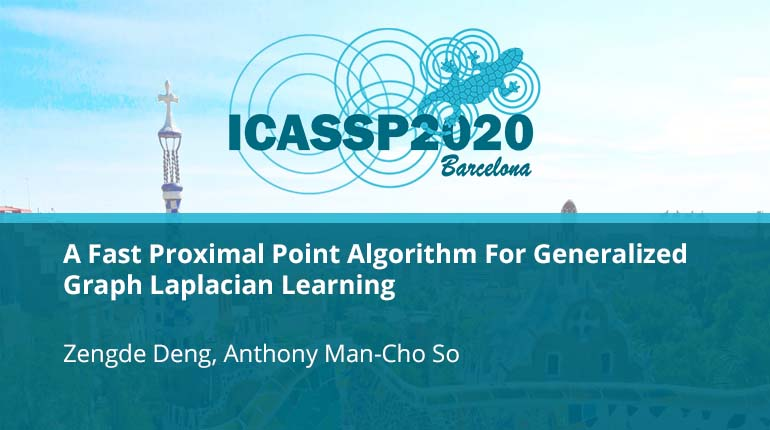# A Fast Proximal Point Algorithm For Generalized Graph Laplacian Learning

Collection:Graph learning is one of the most important tasks in machine learning, statistics and signal processing. In this paper, we focus on the problem of learning the generalized graph Laplacian (GGL) and propose an efficient algorithm to solve it. We first full
• IEEE MemberUS \$11.00
• Society MemberUS \$0.00
• IEEE Student MemberUS \$11.00
• Non-IEEE MemberUS \$15.00
Purchase

## A Fast Proximal Point Algorithm For Generalized Graph Laplacian Learning

Graph learning is one of the most important tasks in machine learning, statistics and signal processing. In this paper, we focus on the problem of learning the generalized graph Laplacian (GGL) and propose an efficient algorithm to solve it. We first full